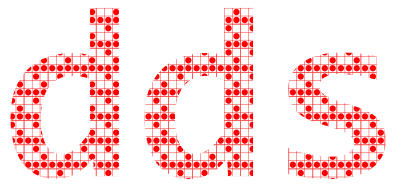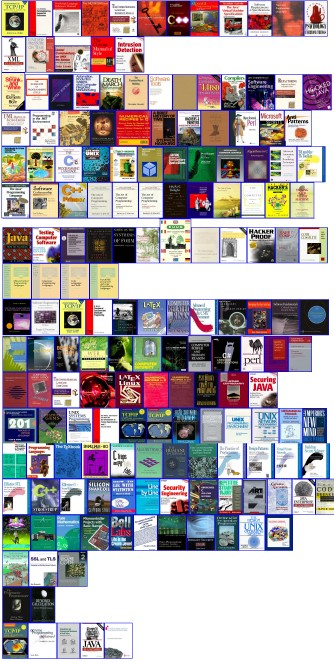Diomidis Spinellis blog## Digital Data Makes Anything Possible

Once data becomes digital anything and everything becomes possible. Consider arranging the books on your bookshelf by the color of their book cover.

The following Perl script this entry does exactly that, using book cover images obtained from amazon.com. The image below is the end-result scaled to 25%. You can see the actual web page here. There are some interesting aspects in the ordering: Knuth's ans Salus's multi-volume works have their volumes appear together; the same is true for four Addison-Wesley titles with a similar cover design. On the other hand, many Prentice-Hall books with the distinctive orange bar on the side, ended up in different rows.```#!/bin/perl
# Order the book cover images by color

\$rows = 15;

print q{<?xml version="1.0" encoding="utf-8" ?>
<!DOCTYPE notes SYSTEM "/pub/schema/notes.dtd">
<notes>
};

@img = (glob('*.jpg'), glob('*.gif'));
for \$f (@img) {
# Skip unavailable images
next if (\$size < 1000 || \$f =~ m/amazon\.gif/);
# Decode image into PNM
if (\$f =~ m/\.jpg/) {
system("djpeg \$f image.pnm");
} else {
}
# Collect color values
open(IN, 'image.pnm') || die;
\$_ = <IN>;
\$_ = <IN>;
(\$x, \$y) = split;
\$_ = <IN>;
\$rsum = \$gsum = \$bsum = 0;
\$n = \$x * \$y;
undef \$data;
for (\$i = 0; \$i < \$n; \$i++) {
(\$r, \$g, \$b) = unpack('CCC', \$rgb);
\$data .= \$rgb;
\$rsum += \$r;
\$gsum += \$g;
\$bsum += \$b;
}
\$rsum /= \$n;
\$gsum /= \$n;
\$bsum /= \$n;
# Skip duplicates
next if (\$seen{\$data});
\$seen{\$data} = 1;
# Calculate saturation and color hue
\$brightness = \$maxcomp = max3(\$rsum, \$gsum, \$bsum);
\$mincomp = min3(\$rsum, \$gsum, \$bsum);
\$saturation = (\$maxcomp - \$mincomp) / \$maxcomp;
if (\$mincomp == \$bsum) {
\$hue = 120 * (\$gsum - \$mincomp) / (\$rsum + \$gsum - 2 * \$mincomp);
} elsif (\$mincomp == \$rsum) {
\$hue = 120 * (1 + \$bsum - \$mincomp) / (\$bsum + \$rsum - 2 * \$mincomp);
} else {
\$hue = 120 * (2 + \$rsum - \$mincomp) / (\$rsum + \$bsum - 2 * \$mincomp);
}
\$row = int(\$hue / 360 * \$rows);
\$brightness{\$f} = \$brightness;
push(@{\$row[\$row]}, \$f);
#print "\$f \$x \$y \$row \$saturation\n";
}

# Print books in color value rows ordered by saturation
for (\$i = 0; \$i < \$rows; \$i++) {
@r = @{\$row[\$i]};
for \$f (sort by_brightness @r) {
\$isbn = \$f;
\$isbn =~ s/\..*//;
print qq{<a href="http://www.amazon.com/exec/obidos/ASIN/\$isbn/dds-20"><img src="\$f" alt="Cover of book with ISBN \$isbn" /></a><!--
-->};
}
print "<br />\n";
}

print q{
<p /> <p /> <p /> <p />
Cover images obtained from
<a href="http://www.amazon.com/exec/obidos/redirect-home/dds-20">
<br />
<img src="amazon.gif" border="0" alt="Amazon.com" /></a><p />
<br />
Some cover images and links are related to the current book edition.
</notes>
};

# Sorting function
sub by_brightness
{
my (\$a, \$b) = @_;

return \$brightness{\$b} <=> \$brightness{\$a};
}

sub max2
{
my (\$a, \$b) = @_;

return \$a > \$b ? \$a : \$b;
}

sub max3
{
my (\$a, \$b, \$c) = @_;

return max2(max2(\$a, \$b), \$c);
}

sub min2
{
my (\$a, \$b) = @_;

return \$a < \$b ? \$a : \$b;
}

sub min3
{
my (\$a, \$b, \$c) = @_;

return min2(min2(\$a, \$b), \$c);
}
```